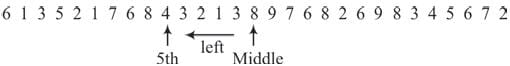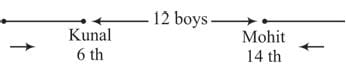# Olympiad Test:Number Ranking - 2

Test Description

## 10 Questions MCQ Test | Olympiad Test:Number Ranking - 2

Olympiad Test:Number Ranking - 2 for Class 8 2023 is part of Class 8 preparation. The Olympiad Test:Number Ranking - 2 questions and answers have been prepared according to the Class 8 exam syllabus.The Olympiad Test:Number Ranking - 2 MCQs are made for Class 8 2023 Exam. Find important definitions, questions, notes, meanings, examples, exercises, MCQs and online tests for Olympiad Test:Number Ranking - 2 below.
Solutions of Olympiad Test:Number Ranking - 2 questions in English are available as part of our course for Class 8 & Olympiad Test:Number Ranking - 2 solutions in Hindi for Class 8 course. Download more important topics, notes, lectures and mock test series for Class 8 Exam by signing up for free. Attempt Olympiad Test:Number Ranking - 2 | 10 questions in 20 minutes | Mock test for Class 8 preparation | Free important questions MCQ to study for Class 8 Exam | Download free PDF with solutions
 1 Crore+ students have signed up on EduRev. Have you?
Olympiad Test:Number Ranking - 2 - Question 1

### Rohit is 14th from the right end in a row of 70 students. What is his position from left end?

Detailed Solution for Olympiad Test:Number Ranking - 2 - Question 1

Numbers of students towards left to Rohit = Rohit’s position from left = 56 + 1 = 57th

Olympiad Test:Number Ranking - 2 - Question 2

### Manak ranks 5th from the top and third seventh from the bottom in a class, How many students are there in the class?

Detailed Solution for Olympiad Test:Number Ranking - 2 - Question 2

Total number of students = 4 + 1 + 36 = 41.

Olympiad Test:Number Ranking - 2 - Question 3

### Which is the 5th number to the left of the number which is exactly in the middle of the given sequence of numbers? 6 1 3 5 21 7 6 8 4 3 2 1 3 8 9 7 6 8 2 6 9 8 3 45 6 7 2

Detailed Solution for Olympiad Test:Number Ranking - 2 - Question 3Total numbers = 29.
Middle number = 15th number

Olympiad Test:Number Ranking - 2 - Question 4

How many numbers from 50 to 150 are there each of which is exactly divisible by 4 and also has 4 as a digit?

Detailed Solution for Olympiad Test:Number Ranking - 2 - Question 4

The numbers divisible by 4 from 50 to 150 are 52, 56, 60, 64, 68, 72, 76, 80, 84, 88, 92, 96, 100,104, 108, 112, 116, 120, 124, 128, 132, 136, 140, 144, 148.
The numbers which has 4 as a digit are 64, 84, 104, 124, 140, l44, 148

Olympiad Test:Number Ranking - 2 - Question 5

How many numbers from 1 to 100 are there which are exactly divisible by 7 but not by 3?

Detailed Solution for Olympiad Test:Number Ranking - 2 - Question 5

The numbers from 1 to 100 which are divisible by 7 but not by 3 are
7, 14, 28, 35, 49, 56, 70, 77, 91, 98,

Olympiad Test:Number Ranking - 2 - Question 6

Ranjit ranked fifteenth from the top and fourty-sixth from the bottom in a class. How many students are there in the class?

Detailed Solution for Olympiad Test:Number Ranking - 2 - Question 6

Total number of students = 14 + 1 + 45 = 60

Olympiad Test:Number Ranking - 2 - Question 7

Sunita ranks fifteenth in a class of 75 students. What is her rank from the last?

Detailed Solution for Olympiad Test:Number Ranking - 2 - Question 7

Number of students behind Sunita in rank = 75 – 15 = 60.
Sunita’s rank from last = 60 + 1 = 61

Olympiad Test:Number Ranking - 2 - Question 8

In a class of 45 students, Raju is 7th from the bottom, where as Samir is placed 9th from the top. Peter is placed exactly in between two. What is Raju’s position from Peter?

Detailed Solution for Olympiad Test:Number Ranking - 2 - Question 8

Number of students between Raju and Samir = 45 – (7 + 9) = 45 – 16 = 29

Peter is 15th in between Raju and Samir. Raju’s position is 15th from Peter.

Olympiad Test:Number Ranking - 2 - Question 9

Kunal is 6th from the left end and Mohit is 14th from the right end in a row of boys. If there are 12 boys between Kunal and Mohit then how many boys are there in the row?

Detailed Solution for Olympiad Test:Number Ranking - 2 - Question 9Total number of boys = 6 + 12 + 14 = 32

Olympiad Test:Number Ranking - 2 - Question 10

In a row of boys if Shankar is 10th from the left and Mahesh is 9th from the right interchange their positions, Shankar becomes15th from the left. How many students are there in the row?

Detailed Solution for Olympiad Test:Number Ranking - 2 - Question 10

Number of students in the row = (15 + 9) – 1 = 24 – 1 = 23

 Use Code STAYHOME200 and get INR 200 additional OFF Use Coupon Code